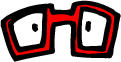# One QuickieConverting RGB to HSL (Graphics->General)
Some color manipulations are easier in HSL than RGB.
```// Based on Foley and van Dam algorithm.
void ConvertRGBToHSL (const CGFloat *rgbComponents, CGFloat *hslComponents) {
CGFloat red = rgbComponents;
CGFloat green = rgbComponents;
CGFloat blue = rgbComponents;

CGFloat maxColor = MAX (red, MAX (green, blue));
CGFloat minColor = MIN (red, MIN (green, blue));
CGFloat deltaColor = maxColor - minColor;

CGFloat hue, saturation;
CGFloat lightness =  (maxColor + minColor) / 2.0;

// All the same means a gray color.
if (maxColor == minColor) {
saturation = 0.0;
hue = 0.0;  // officially undefined with gray, but go ahead and zero out.

} else {
if (lightness < 0.5) saturation = deltaColor / (maxColor + minColor);
else                 saturation = deltaColor / (2.0 - deltaColor);

if (red == maxColor)        hue = (green - blue) / deltaColor;
else if (green == maxColor) hue = 2.0 + (blue - red) / deltaColor;
else                        hue = 4.0 + (red - green) / deltaColor;

// H will be in the range of 0..6, convert to degrees.
hue *= 60.0;

// Convert to positive angle.
if (hue < 0) hue += 360.0;
}

// Clamp to legal values.
hslComponents = MAX (0.0, MIN (360.0, hue));
hslComponents = MAX (0.0, MIN (1.0, saturation));
hslComponents = MAX (0.0, MIN (1.0, lightness));

} // ConvertRGBToHSL```

borkware home | products | miniblog | rants | quickies | cocoaheads
Advanced Mac OS X Programming book

webmonster@borkware.com